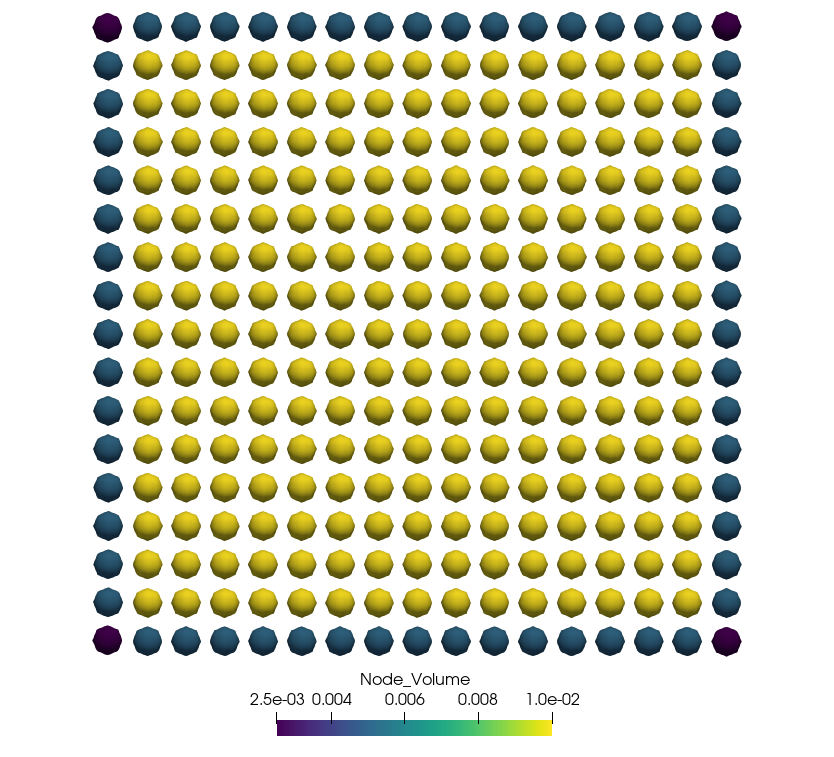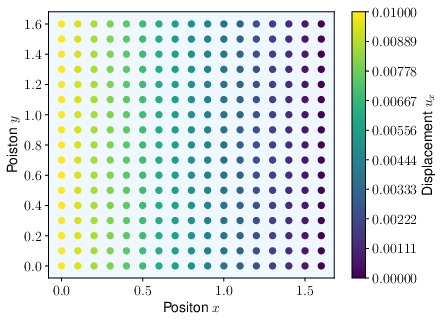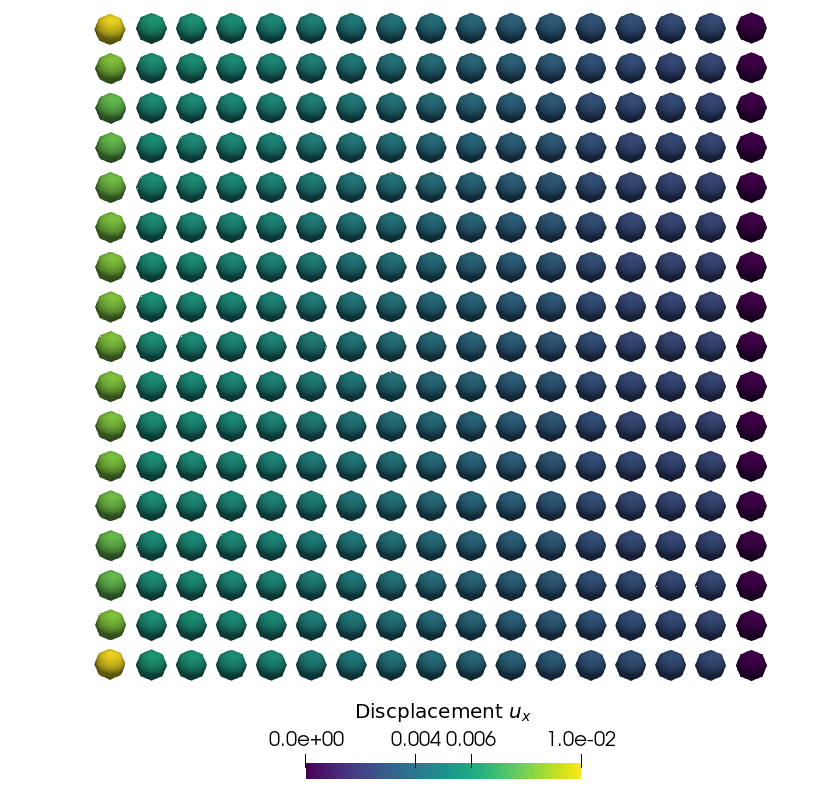## Generating the mesh

The file input_mesh.yaml in the example folder will generate the mesh as shown in the following figure.## Model

The quasi-static models as described in  is used to assemble the tangent stiffness matrix and obtain the solution by solving Newton steps.

Model:
Dimension: 2
Discretization_Type:
Spatial: finite_difference
Time: quasi_static
Final_Time: 1
Time_Steps: 1
Horizon: 0.5
Horizon_h_Ratio: 5


### Material model

The state-based elastic material models as described in  is implemented for the ElasticState material.

Material:
Type: ElasticState
Density: 1200
Compute_From_Classical: true
K: 4000.0
G: 1500.0
Is_Plane_Strain: False
Influence_Function:
Type: 1


### Applying boundary conditions

#### Displacement boundary conditions

The following code applies a fixed displacement to the first layer of nodes on the right-hand side in the first figure in both directions.

Displacement_BC:
Sets: 2
Set_1:
Location:
Line: [1.55, 1.65]
Direction: 
Time_Function:
Type: constant
Parameters:
- 0.0
Spatial_Function:
Type: constant
Set_2:
Location:
Line: [1.55, 1.65]
Direction: 
Time_Function:
Type: constant
Parameters:
- 0.0
Spatial_Function:
Type: constant



#### Force boundary conditions

The following code applies a external body force density to the last node on the left-hand side in the first figure.

Note that in this example we want to apply a force $F=-40N$, however, the body force density has the units $\frac{N}{mm^2}$. Thus, the force is devided by the area $1.6\times 0.1$, where $1.6$ is the length of the plate and $0.1$ is the mesh width. This results in a body force density $-250$.

Force_BC:
Sets: 1
Set_1:
Location:
Line: [-0.1, 0.05]
Direction: 
Time_Function:
Type: linear
Parameters:
- 1
Spatial_Function:
Type: constant
Parameters:
- -250.0


### Solver

Solver:
Type: BiCGSTAB
Max_Iteration: 200
Tolerance: 1e-6
Perturbation: 1e-7


## Validation

The prediction of the displacement field in $x$-direction is shown in second figure. For the derivation of the displacement field, we refer to .The third figure shows the obtained displacement field by the code using the above configuration.1. Littlewood, David J. “Roadmap for peridynamic software implementation.” SAND Report, Sandia National Laboratories, Albuquerque, NM and Livermore, CA (2015).
2. Silling, Stewart A., et al. “Peridynamic states and constitutive modeling.” Journal of Elasticity 88.2 (2007): 151-184. 3.